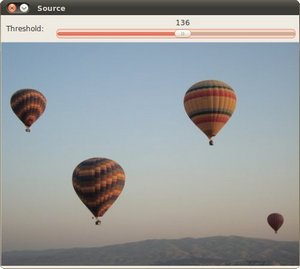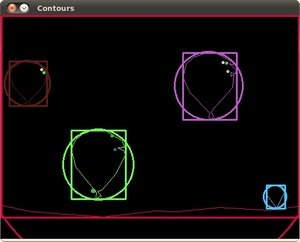OpenCV  3.4.0 Open Source Computer Vision
Creating Bounding boxes and circles for contours

## Goal

In this tutorial you will learn how to:

## Code

This tutorial code's is shown lines below. You can also download it from here

#include <iostream>
using namespace cv;
using namespace std;
Mat src; Mat src_gray;
int thresh = 100;
int max_thresh = 255;
RNG rng(12345);
void thresh_callback(int, void* );
int main( int argc, char** argv )
{
CommandLineParser parser( argc, argv, "{@input | ../data/stuff.jpg | input image}" );
if( src.empty() )
{
cout << "Could not open or find the image!\n" << endl;
cout << "usage: " << argv << " <Input image>" << endl;
return -1;
}
cvtColor( src, src_gray, COLOR_BGR2GRAY );
blur( src_gray, src_gray, Size(3,3) );
const char* source_window = "Source";
namedWindow( source_window, WINDOW_AUTOSIZE );
imshow( source_window, src );
createTrackbar( " Threshold:", "Source", &thresh, max_thresh, thresh_callback );
thresh_callback( 0, 0 );
waitKey(0);
return(0);
}
void thresh_callback(int, void* )
{
Mat threshold_output;
vector<vector<Point> > contours;
vector<Vec4i> hierarchy;
threshold( src_gray, threshold_output, thresh, 255, THRESH_BINARY );
findContours( threshold_output, contours, hierarchy, RETR_TREE, CHAIN_APPROX_SIMPLE, Point(0, 0) );
vector<vector<Point> > contours_poly( contours.size() );
vector<Rect> boundRect( contours.size() );
vector<Point2f>center( contours.size() );
for( size_t i = 0; i < contours.size(); i++ )
{
approxPolyDP( contours[i], contours_poly[i], 3, true );
boundRect[i] = boundingRect( contours_poly[i] );
}
Mat drawing = Mat::zeros( threshold_output.size(), CV_8UC3 );
for( size_t i = 0; i< contours.size(); i++ )
{
Scalar color = Scalar( rng.uniform(0, 255), rng.uniform(0,255), rng.uniform(0,255) );
drawContours( drawing, contours_poly, (int)i, color, 1, 8, vector<Vec4i>(), 0, Point() );
rectangle( drawing, boundRect[i].tl(), boundRect[i].br(), color, 2, 8, 0 );
circle( drawing, center[i], (int)radius[i], color, 2, 8, 0 );
}
namedWindow( "Contours", WINDOW_AUTOSIZE );
imshow( "Contours", drawing );
}

## Explanation

The main function is rather simple, as follows from the comments we do the following:

1. Open the image, convert it into grayscale and blur it to get rid of the noise.
CommandLineParser parser( argc, argv, "{@input | ../data/stuff.jpg | input image}" );
if( src.empty() )
{
cout << "Could not open or find the image!\n" << endl;
cout << "usage: " << argv << " <Input image>" << endl;
return -1;
}
cvtColor( src, src_gray, COLOR_BGR2GRAY );
blur( src_gray, src_gray, Size(3,3) );
2. Create a window with header "Source" and display the source file in it.
const char* source_window = "Source";
namedWindow( source_window, WINDOW_AUTOSIZE );
imshow( source_window, src );
3. Create a trackbar on the source_window and assign a callback function to it In general callback functions are used to react to some kind of signal, in our case it's trackbar's state change.
createTrackbar( " Threshold:", "Source", &thresh, max_thresh, thresh_callback );
4. Explicit one-time call of thresh_callback is necessary to display the "Contours" window simultaniously with the "Source" window.
thresh_callback( 0, 0 );
5. Wait for user to close the windows.
waitKey(0);

The callback function thresh_callback does all the interesting job.

1. Writes to threshold_output the threshold of the grayscale picture (you can check out about thresholding here).
threshold( src_gray, threshold_output, thresh, 255, THRESH_BINARY );
2. Finds contours and saves them to the vectors contour and hierarchy.
findContours( threshold_output, contours, hierarchy, RETR_TREE, CHAIN_APPROX_SIMPLE, Point(0, 0) );
3. For every found contour we now apply approximation to polygons with accuracy +-3 and stating that the curve must me closed.

After that we find a bounding rect for every polygon and save it to boundRect.

At last we find a minimum enclosing circle for every polygon and save it to center and radius vectors.

for( size_t i = 0; i < contours.size(); i++ )
{
approxPolyDP( contours[i], contours_poly[i], 3, true );
boundRect[i] = boundingRect( contours_poly[i] );
}

We found everything we need, all we have to do is to draw.

4. Create new Mat of unsigned 8-bit chars, filled with zeros. It will contain all the drawings we are going to make (rects and circles).
Mat drawing = Mat::zeros( threshold_output.size(), CV_8UC3 );
5. For every contour: pick a random color, draw the contour, the bounding rectangle and the minimal enclosing circle with it,
for( size_t i = 0; i< contours.size(); i++ )
{
Scalar color = Scalar( rng.uniform(0, 255), rng.uniform(0,255), rng.uniform(0,255) );
drawContours( drawing, contours_poly, (int)i, color, 1, 8, vector<Vec4i>(), 0, Point() );
rectangle( drawing, boundRect[i].tl(), boundRect[i].br(), color, 2, 8, 0 );
circle( drawing, center[i], (int)radius[i], color, 2, 8, 0 );
}
6. Display the results: create a new window "Contours" and show everything we added to drawings on it.
namedWindow( "Contours", WINDOW_AUTOSIZE );
imshow( "Contours", drawing );

## Result

Here it is: